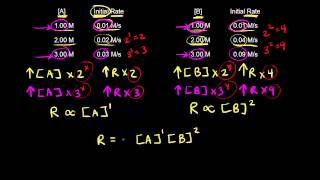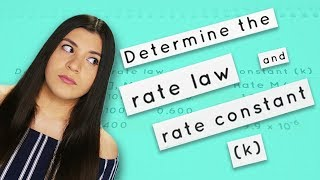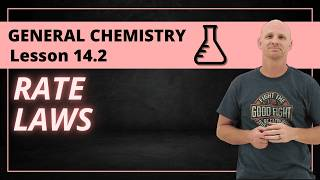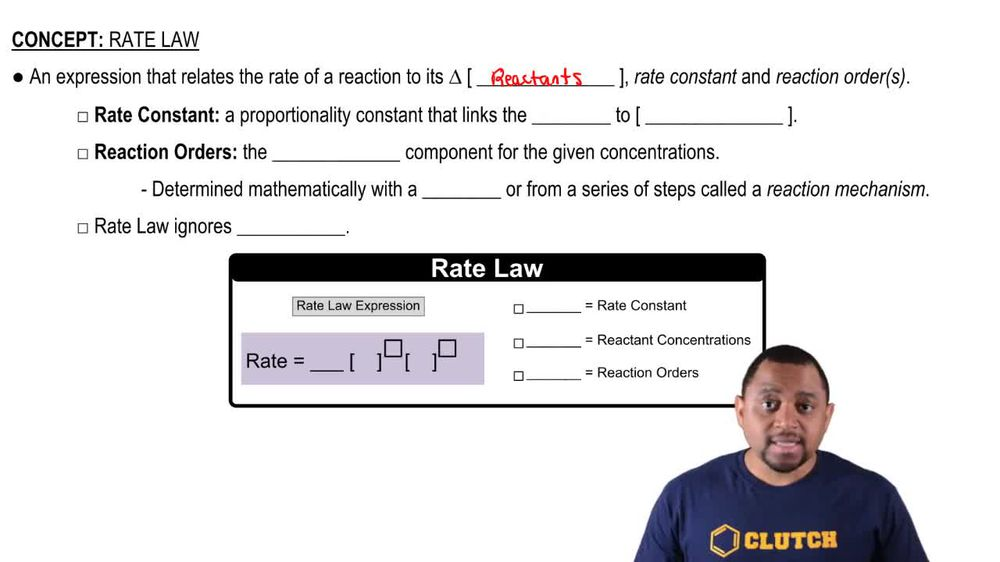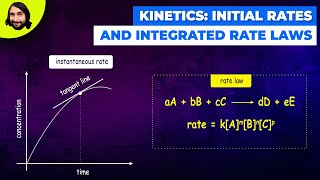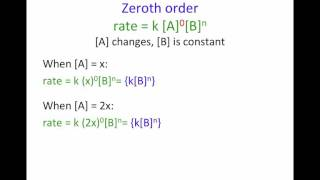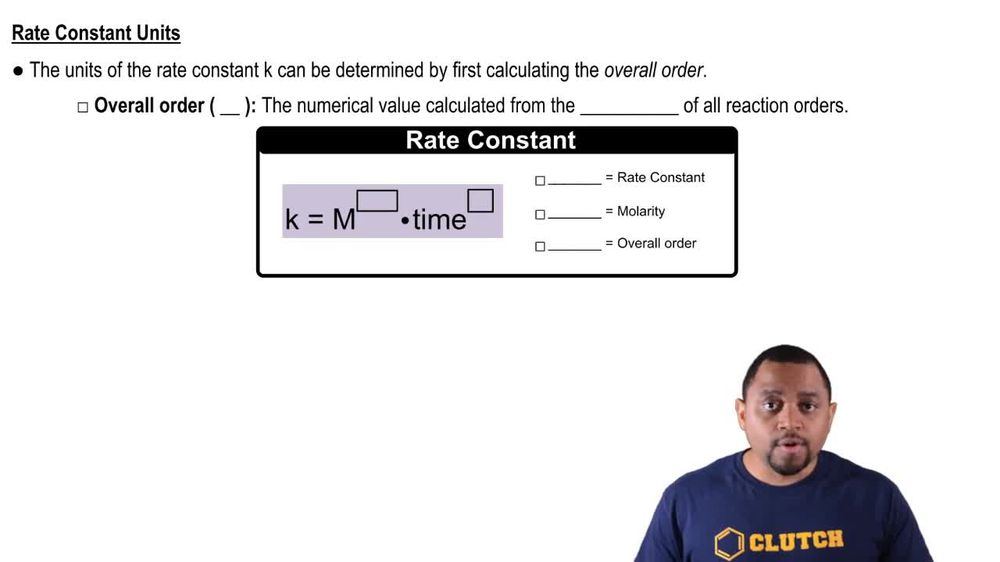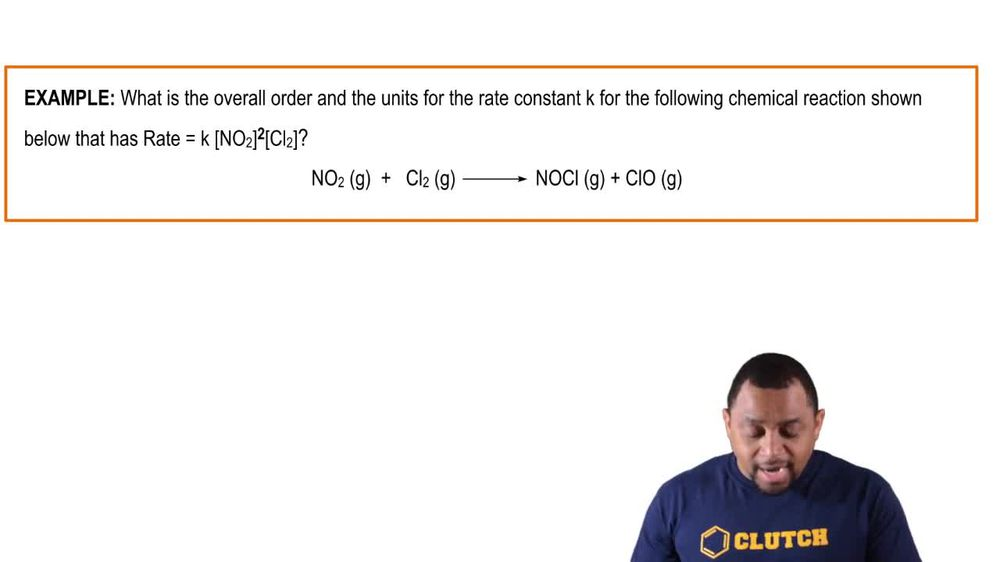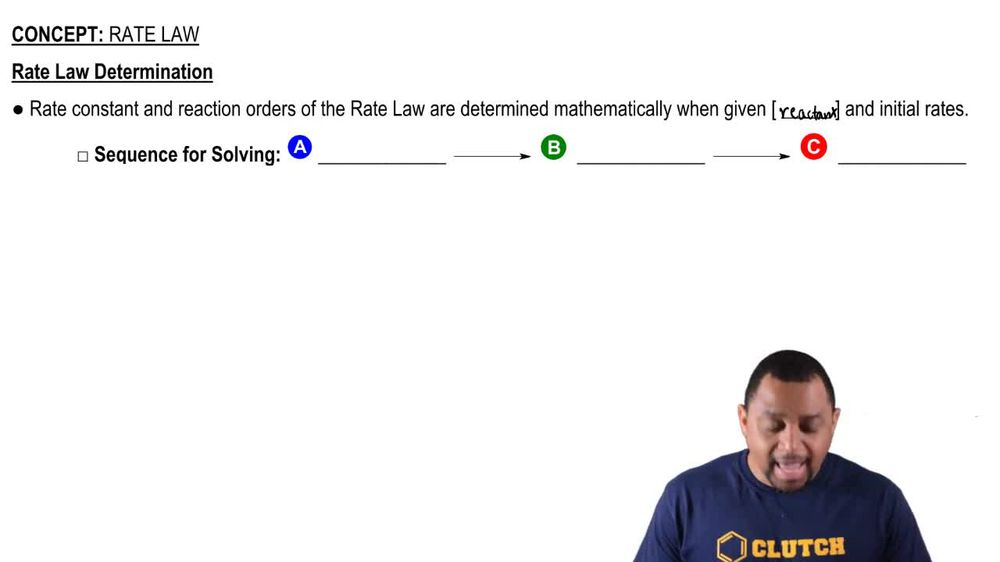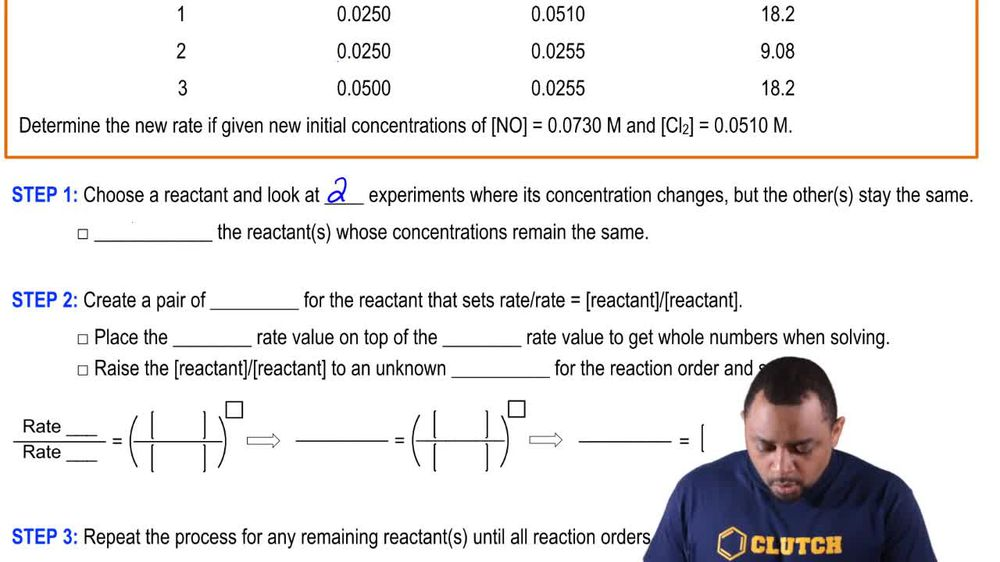Start typing, then use the up and down arrows to select an option from the list.
1. 15. Chemical Kinetics2. Rate Law# Rate Law Example 1

by Jules Bruno
150 views
0
the chemical reaction has a rate law of K. Eight of the three B. To the one C. To the zero. By what factor would the rate increase if the concentration of A. Were tripled, the concentration of B. Was cut by half and the concentration of C. Was increased by half and the rate constant K. Was kept constant. Alright so here we're going to say rate equals K. eight of the three B. to the one c. to the zero. They're telling me that our rick constant K. Staying the same. It's not changing so it's not going to affect any change in rate so we can ignore it for now and here we're talking about tripling, cutting in half, increasing by half each one of these reactant concentrations to make it easy for us. They don't give us their initial amounts so just make them all one molar. Okay? Just to make it easy because proportionately it wouldn't matter. I can make them all three moller doing what it tells me to do, I still get the same answer. So here we're going to assume that they're all starting at one molar. And here I'm going to triple a. It's going to go from one molar to three molar And it's still to the three B. Is getting cut by half. So you start at one moller, you cut it by half so now you only have 10.5 left Still to the one And see we're still gonna do. See but it's not gonna really matter, we're increasing it by half so that it becomes 1.5 C really doesn't matter because any number to the zeroth power is equal to one so I can make this concentration inside for C a million. It won't matter because a million to zero is still equal to one. So really all that is important to figure out. The changing rate is A. And B. So three to the three is 27 Times . is 13.5. This means that my rate would be expected to increase by 13.5 fold. This is how much faster my rate would go because increasing the concentration of my reactant would cause an increase in the rate. Here we're seeing it mathematically being shown to us. So here option C would be the correct answer.